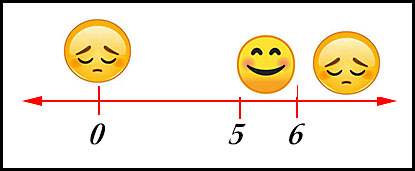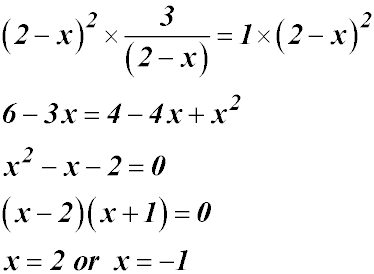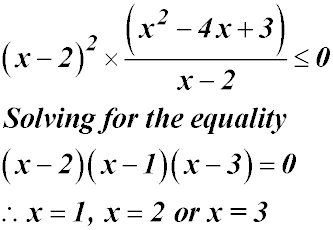Dr. J's Maths.
Where the techniques of Maths
are explained in simple terms.

Algebra - Inequalities - Inequalities - variable in the denominator.
Test Yourself 1 - Solutions.

 Multiplication can create an x2 term.Hence the required domain is either: between 5 and 6 or less than 5 and greater than 6 Test a number in the original inequality - 0 is easy and it is in the second domain above. We get 0 after substitution which is less than 3 - so the inequality is not satisfied (sad).Hence the required domain is from 5 to 6. But the correct domain cannot include 5 because then the denominator would be 0 - not generally a good thing!!! So our solution is 5 < x ≤ 6.Hence the required domain is either: between -1 and 2 or less than -1 and greater than 2. Test a number in the original inequality - 0 is easy and it is in the first domain above. We get 1.5 after substitution which is greater than 1 - so the inequality is satisfied (so be happy).Hence the required domain is from -1 to 2. The correct domain cannot include 2 because then the denominator would be 0 - so the fraction would be undefined!!! So our solution is -1 ≤ x < 2.Multiplication can create an term greater than x2.Hence the required domain is either: between 1 and 2 or greater than 3; less than 1 or between 2 and 3.Test a number in the original inequality - 0 is easy and it is in the second domain above. We get -1.5 after substitution into the left side which is less than 0 - so the inequality is satisfied (happy). So our solution is x ≤ 1 or -2 ≤ x ≤ 3.Where on the graph of y = loge (x-2) is the gradient of the curve greater than 1.